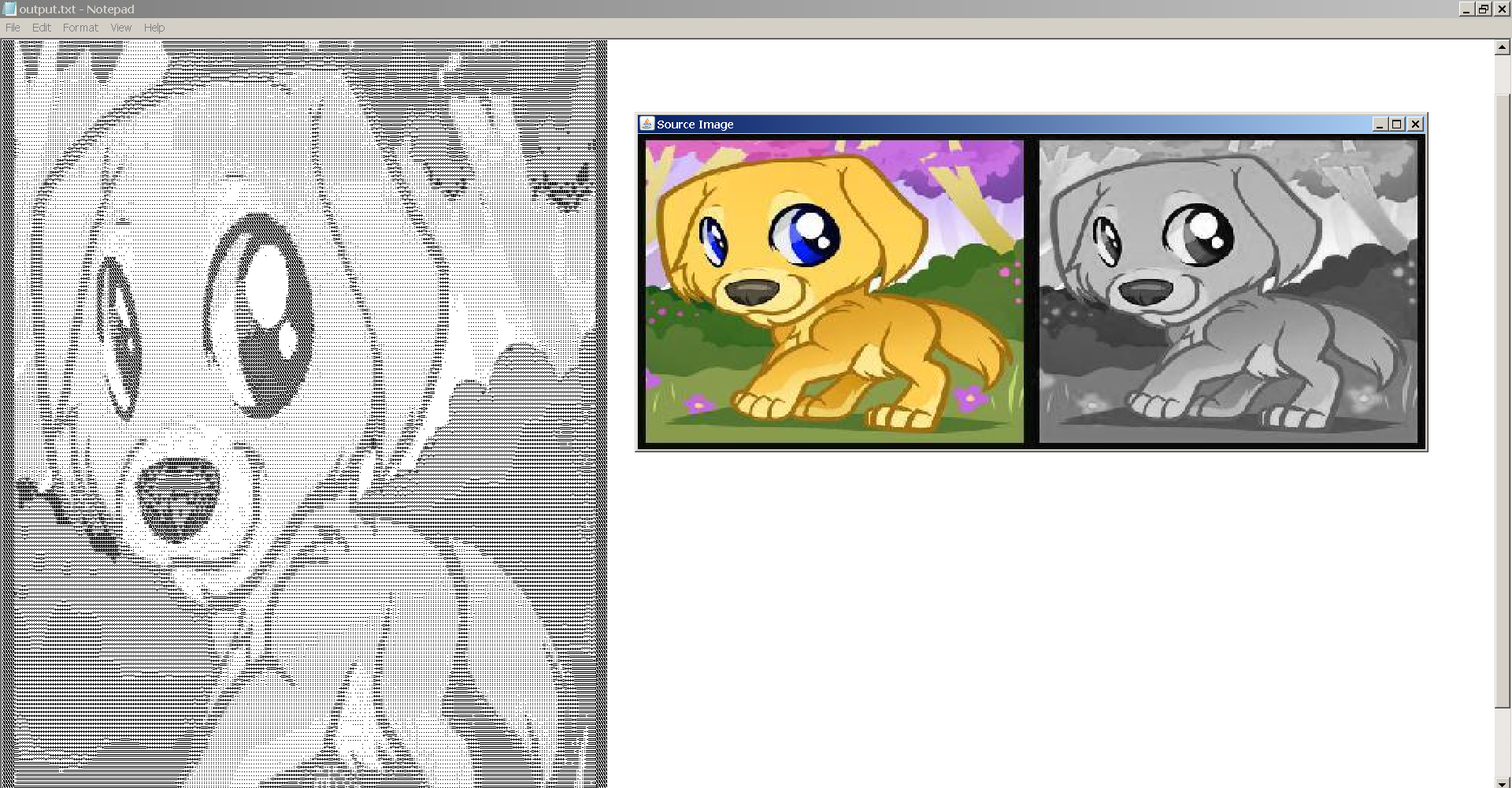## ASCII Art Converter

This was originally posted on February 16, 2016 and was rewritten and reuploaded on October 13, 2019

In the February Code Dojo, Jemma and I made an ASCII art converter in Java that takes an image as input and converts it into a text document which, when zoomed out far enough, imitates the original image.From left to right, the output text document, the input image, and the input image converted to greyscale.

This was done by first iterating over every pixel in the image after it has been onverted to greyscale. The luminance of every pixel was then calculated by using a formula found online:

```                ```// calc luminance in range 0.0 to 1.0; using SRGB luminance constants
float luminance = (red * 0.2126f + green * 0.7152f + blue * 0.0722f) / 255;
```
```

This luminance value was then stored in a very large 2D array. The array is then looped over and certain ASCII characters are chosen based on the luminance value selected.

`diffLum` is the difference between the largest value of luminance found (largeLum) and the smallest value of luminance found (smallLum), the addition of this as opposed to set values between 0 and 1.0 mean that the whole range of characters is still used even if there is not a great difference in the source image's luminance values.

```                ```// choose brightness threshold as appropriate:
if (luminance <= (diffLum*0.1) + smallLum) {
chars[x][y] = ' ';
} else if (luminance <= (diffLum*0.2) + smallLum) {
chars[x][y] = '.';
} else if (luminance <= (diffLum*0.3) + smallLum) {
chars[x][y] = ':';
} else if (luminance <= (diffLum*0.4) + smallLum) {
chars[x][y] = '-';
} else if (luminance <= (diffLum*0.5) + smallLum) {
chars[x][y] = '=';
} else if (luminance <= (diffLum*0.6) + smallLum) {
chars[x][y] = '+';
} else if (luminance <=(diffLum*0.7) + smallLum) {
chars[x][y] = '*';
} else if (luminance <= (diffLum*0.8) + smallLum) {
chars[x][y] = '#';
} else if (luminance <= (diffLum*0.9) + smallLum) {
chars[x][y] = '%';
} else {
chars[x][y] = '@';
}
```
```

The chars array is then looped over and written into a text, .txt, file.

The solution works adequately however the output cannot be resized and so the source image must be downsized quite a bit before it is truly useful as an ASCII converter.